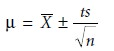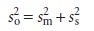Home | | Modern Analytical Chemistry | The Importance of Sampling

# The Importance of Sampling

When a manufacturer produces a chemical they wish to list as ACS Reagent Grade, they must demonstrate that it conforms to specifications established by the Ameri- can Chemical Society (ACS).

The Importance of Sampling

When a manufacturer produces a chemical they wish to list as ACS Reagent Grade, they must demonstrate that it conforms to specifications established by the Ameri- can Chemical Society (ACS). For example, ACS specifications for NaHCO3 require that the concentration of iron be less than or equal to 0.001% w/w. To verify that a production lot meets this standard, the manufacturer performs a quantitative analy- sis, reporting the result on the productâ€™s label. Because it is impractical to analyze the entire production lot, its properties are estimated from a limited sampling. Sev- eral samples are collected and analyzed, and the resulting mean, Xâ€“, and standard de- viation, s, are used to establish a confidence interval for the production lotâ€™s true mean, ÎĽwhere n is the number of samples, and t is a statistical factor whose value is deter- mined by the number of samples and the desired confidence level.*

Selecting a sample introduces a source of determinate error that cannot be cor- rected during the analysis. If a sample does not accurately represent the population from which it is drawn, then an analysis that is otherwise carefully conducted will yield inaccurate results. Sampling errors are introduced whenever we extrapolate from a sample to its target population. To minimize sampling errors we must col- lect the right sample.

Even when collecting the right sample, indeterminate or random errors in sam- pling may limit the usefulness of our results. Equation 7.1 shows that the width of a confidence interval is directly proportional to the standard deviation.

The overall standard deviation for an analysis, so, is determined by ran- dom errors affecting each step of the analysis. For convenience, we di- vide the analysis into two steps. Random errors introduced when collect- ing samples are characterized by a standard deviation for sampling, ss. The standard deviation for the analytical method, sm, accounts for ran- dom errors introduced when executing the methodâ€™s procedure. The re- lationship among so, ss, and sm is given by a propagation of  random errorEquation 7.2 shows that an analysisâ€™ overall variance may be lim- ited by either the analytical method or sample collection. Unfortu- nately, analysts often attempt to minimize overall variance by im- proving only the methodâ€™s precision. This is futile, however, if the standard deviation for sampling is more than three times greater than that for the method. Figure 7.1 shows how the ratio sm/ss affects the percentage of overall variance attributable to the method. When the methodâ€™s standard deviation is one third of that for sampling, indeterminate method errors explain only 10% of the overall variance. Attempting to improve the analysis by decreasing sm provides only a nominal change in the overall variance.To determine which step has the greatest effect on the overall variance, both sm2 and s2 must be known. The analysis of replicate samples can be used to estimate the overall variance. The variance due to the method is determined by analyzing a stan- dard sample, for which we may assume a negligible sampling variance. The variance due to sampling is then determined by difference.Study Material, Lecturing Notes, Assignment, Reference, Wiki description explanation, brief detail
Modern Analytical Chemistry: Obtaining and Preparing Samples for Analysis : The Importance of Sampling |